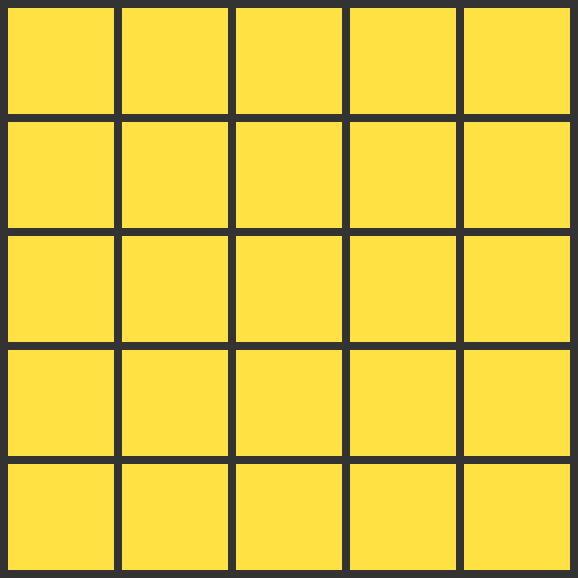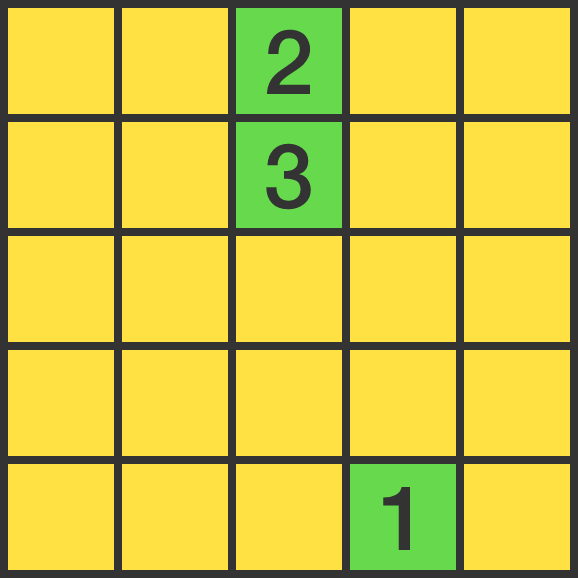# Go ${\color{#20A900} \mathbf{green}}$!

Logic Level 3You paint the $5 \times 5$ grid green, one square at a time. You can only paint a square green if it shares an edge with 1 or 3 yellow squares (diagonals don't count).

What's the maximum number of squares you can color?

Below right is an example of a sequence of how you might paint the first 3 squares.Clarification: The square colored green with sequence number 2 now borders two yellow squares, but it was bordering three yellow squares at the time of coloring.

×